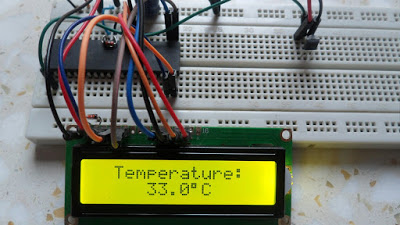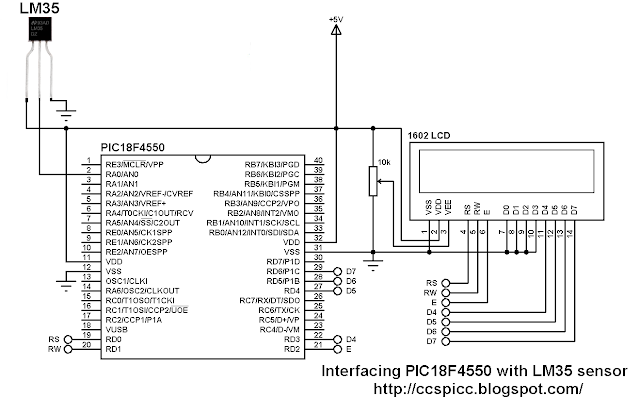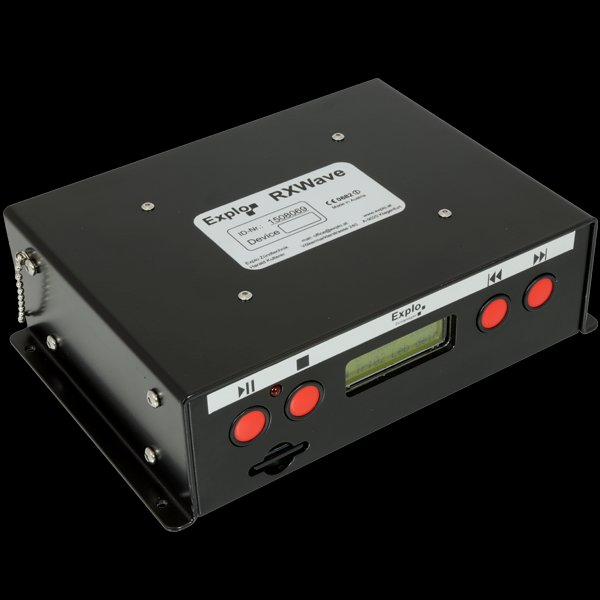# Interfacing LM35 temperature sensor with PIC18F4550 microcontroller

Interfacing PIC18F4550 with LM35

This small topic shows the circuit diagram and CCS C code of the interfacing of LM35 temperature sensor with PIC18F4550 microcontroller.The LM35 temperature sensor is three pin device (VCC, OUT and GND) with an output voltage linearly related to Centigrade temperature. Since the LM35 output varies with dependent to the temperature we need ADC (Analog-to-Digital Converter) module to measure this voltage. The ADC module converts analog data into digital data.
The LM35 output has linear +10mV/°C scale factor means the following:
If the output voltage =   10mV —> temperature =   1°C
If the output voltage = 100mV —> temperature = 10°C
If the output voltage = 200mV —> temperature = 20°C
If the output voltage = 370mV —> temperature = 37°C
and so on.
LM35 Futures (from datasheet):

• Calibrated Directly in ° Celsius (Centigrade)
• Linear + 10 mV/°C Scale Factor
• 0.5°C Ensured Accuracy (at +25°C)
• Rated for Full −55°C to +150°C Range
• Suitable for Remote Applications
• Low Cost Due to Wafer-Level Trimming
• Operates from 4 to 30 V
• Less than 60-μA Current Drain
• Low Self-Heating, 0.08°C in Still Air
• Nonlinearity Only ±¼°C Typical
• Low Impedance Output, 0.1 Ω for 1 mA Load

Hardware Required:

• PIC18F4550 microcontroller
• LM35 temperature sensor  — datasheet
• 1602 LCD screen
• 10K ohm variable resistor
• 5V voltage source
• Jumper wires

Interfacing PIC18F4550 with LM35 sensor circuit:

The output of the LM35 temperature sensor is connected to analog channel 0 (AN0) of the PIC18F4550 microcontroller.
In this example the MCU uses its internal oscillator and MCLR pin function is disabled.
Interfacing PIC18F4550 with LM35 temperature sensor C code:
The C code below was tested with CCS PIC C compiler version 5.051.
Reading voltage quantity using the ADC gives us a number between 0 and 1023 (10-bit resolution), 0V is represented by 0 and 5V is represented by 1023. Converting back the ADC digital value is easy and we can use the following equation for that conversion:The output of the LM35 temperature sensor is connected to analog channel 0 (AN0) of the PIC18F4550 microcontroller.
In this example the MCU uses its internal oscillator and MCLR pin function is disabled.
Interfacing PIC18F4550 with LM35 temperature sensor C code:
The C code below was tested with CCS PIC C compiler version 5.051.
Reading voltage quantity using the ADC gives us a number between 0 and 1023 (10-bit resolution), 0V is represented by 0 and 5V is represented by 1023. Converting back the ADC digital value is easy and we can use the following equation for that conversion:
Multiplying the previous result by 100 (LM35 scale factor is 10mV/°C = 0.01V/°C) will gives the actual temperature:
where 0.489 = 500 / 1023
The complete C code is the one below.

```/* Interfacing PIC18F4550 with LM35 analog temperature sensor CCS C code.
Read LM35 datasheet to understand the code!
http://ccspicc.blogspot.com/
[email protected]
*/

//LCD module connections
#define LCD_RS_PIN      PIN_D0
#define LCD_RW_PIN      PIN_D1
#define LCD_ENABLE_PIN  PIN_D2
#define LCD_DATA4       PIN_D3
#define LCD_DATA5       PIN_D4
#define LCD_DATA6       PIN_D5
#define LCD_DATA7       PIN_D6
//End LCD module connections

#include <18F4550.h>
#fuses NOMCLR, INTRC_IO
#use delay(clock = 8MHz)
#include <lcd.c>

char temperature[] = " 00.0 C";
unsigned int16 temp;
void main(){
setup_oscillator(OSC_8MHZ);                    // Set internal oscillator to 8MHz
setup_adc_ports(AN0);                          // Configure AN0 pin as analog
set_adc_channel(0);                            // Select channel 0 (AN0)
lcd_init();                                    // Initialize LCD module
lcd_putc('\f');                                // Clear LCD
lcd_gotoxy(3, 1);                              // Go to column 3 row 1
printf(lcd_putc, "Temperature:");
temperature  = 223;                         // Put degree symbol (°)
while(TRUE){
delay_ms(1000);
temp = read_adc() * 0.489;                   // Read analog voltage and convert it to degree celsius (0.489 = 500/1023)
if (temp > 99)
temperature  = 1 + 48;                  // Put 1 (of hundred)
else
temperature  = ' ';                     // Put space
temperature  = (temp / 10) % 10  + 48;
temperature  =  temp % 10  + 48;
lcd_gotoxy(5, 2);                            // Go to column 5 row 2
printf(lcd_putc, temperature);               // Display LM35 temperature result
}
}
```

The resultSource: Interfacing LM35 temperature sensor with PIC18F4550 microcontroller

#### Current Project / Post can also be found using:

• temperature sensors for use with pic18f4550
• temperature controller LMAO 35 with pic 18f4550 on protus with sdmemory soft ware
• interfacing of temp sensor with microcontroller